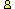CTK Exchange CTK Wiki Math Front Page Movie shortcuts Personal info Awards Terms of use Privacy Policy Cut The Knot! MSET99 Talk Games & Puzzles Arithmetic/Algebra Geometry Probability Eye Opener Analog Gadgets Inventor's Paradox Did you know?... Proofs Math as Language Things Impossible My Logo Math Poll Other Math sit's Guest book News sit's Recommend this siteCTK Exchange

 Subject: "Arcsin as different of two radicals" Previous Topic | Next Topic
 ConferencesThe CTK ExchangeThis and thatTopic #956Printer-friendly copyEmail this topic to a friend Reading Topic #956
jmolokach
Member since Aug-17-10
Nov-09-10, 01:20 PM (EST)"Arcsin as different of two radicals"

 In studying for upcoming topics for precalculus course, I was venturing into the ideas of the sum and difference formulas for sine, cosine, and tangent. I stumbled upon this graph:f(x) = sqrt(1+x) - sqrt(1-x) and noticed it to be eerily similar to g(x) = arcsin(x)I noticed the graphs to be a tad bit different so then I wondered if there might be some solution a for which:sqrt(a+x) - sqrt(a-x) = arcsin(x)I do not believe this to be possible but was wondering if someone might comment on why the graphs are so similar. A proof that the above equation is not solvable might find calculus useful I think (I am thinking concavity has something to do with it).What about other roots... say cube root or 4th root or 1.5 root, etc...?Is is preposterous to think that the arcsin function can be written as the difference of two radicals? Or did I just stumble upon a near coincidence?molokach

alexbCharter Member
2655 posts
Nov-09-10, 01:32 PM (EST)1. "RE: Arcsin as different of two radicals"
In response to message #0

 >f(x) = sqrt(1+x) - sqrt(1-x) and noticed it to be eerily >similar to g(x) = arcsin(x) The Maclaurin series of f is x + x^3/8 + ...That of g isx + x^3/6 + ...>I noticed the graphs to be a tad bit different so then I >wondered if there might be some solution a for which: >>sqrt(a+x) - sqrt(a-x) = arcsin(x) I'd guess, with a ≠1, the proximity of the two series would rather worsen because of the loss of symmetry. For a = 1, both functions arer odd.

 Conferences | Forums | Topics | Previous Topic | Next Topic
 Select another forum or conference Lobby The CTK Exchange (Conference)   |--Early math (Public)   |--Middle school (Public)   |--High school (Public)   |--College math (Public)   |--This and that (Public)   |--Guest book (Protected)   |--Thoughts and Suggestions (Public) Educational Press (Conference)   |--No Child Left Behind (Public)   |--Math Wars (Public)   |--Mathematics and general education (Public)You may be curious to have a look at the old CTK Exchange archive.